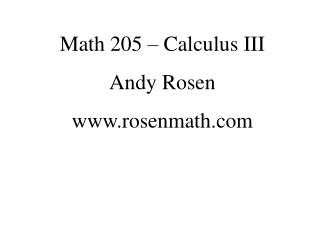Download PresentationMath 205 – Calculus III Andy Rosen rosenmath

Math 205 – Calculus III Andy Rosen rosenmath - PowerPoint PPT Presentation

Math 205 – Calculus III Andy Rosen www.rosenmath.com. Student Learning Outcomes. Students will be able to p erform calculus on vector valued functions. p erform vector operations using geometry in space. p erform calculus on multivariable functions. Vectors.I am the owner, or an agent authorized to act on behalf of the owner, of the copyrighted work described.
Download PresentationMath 205 – Calculus III Andy Rosen rosenmath

Download Policy: Content on the Website is provided to you AS IS for your information and personal use and may not be sold / licensed / shared on other websites without getting consent from its author.While downloading, if for some reason you are not able to download a presentation, the publisher may have deleted the file from their server.

- - - - - - - - - - - - - - - - - - - - - - - - - - E N D - - - - - - - - - - - - - - - - - - - - - - - - - -
Presentation Transcript
1. Math 205 – Calculus III Andy Rosen www.rosenmath.com

2. Student Learning Outcomes Students will be able to • perform calculus on vector valued functions. • perform vector operations using geometry in space. • perform calculus on multivariable functions.

3. Vectors Def. A vector is a quantity that has both magnitude and direction. v is displacement vector from A to B A is the initial point, B is the terminal point v

4. Note that position was not used to determine a vector… v and u are equivalent vectors v = u

5. The vector from (0,0) to (3,4) can be written in component form Because position doesn’t matter, each of these vectors is equivalent The vector representation that has an initial point at (0,0) is in standard position

6. Ex. Find the vector represented by the directed line segment with initial point A(2,-3,4) and terminal point B(-2,1,1).

7. Ex. Consider vector v from (0,0) to (3,2) and vector u from (1,2) to (4,4). Show that u and v are equivalent vectors.

8. Def. The magnitude of a vector is the distance between initial and terminal points. Thm. The length of vector is The length of vector is

9. Def. If u and v are positioned so that the terminal point of u is at the initial point of v, then u + v is the vector with the initial point of u and the terminal point of v.

10. Def. If c is a scalar (non-vector) and v is a vector, then cv is the vector with the same direction as v that has length c times as long as v. If c < 0, then cv goes in the opposite direction as v.

11. u – v = u + (-v)

12. Ex. Given vectors a and b, draw 2a – b.

13. Adding and scalar multiplication numerically: • When adding vectors in component form, add corresponding components • When multiplying by a scalar, multiply each component by the scalar Note that we haven’t talked about multiplying two vectors

14. Ex. Let , find • ||a|| • a + b • 2a – 5b

15. Def. A unit vector is a vector whose length is 1. The unit vector in the direction of a is Ex. Find the unit vector in the direction of

16. Ex. Find a vector in the direction of that has magnitude 7.

17. NoteV2 means the set of all vectors in 2-D, V3 is the set of all vectors in 3-D There are three basic vectors in V3 that we should talk about: i = j = k = • These are the standard basis vectors because they are unit vectors in each of the three dimensions

18. Every vector in V3 can be described using i, j, and k 4i – 2j + k Ex. If a = i + 2j – 3k and b = 4i + 7k, find 2a + 3b.

19. We can also find the vector if we know magnitude and angle: Ex. The magnitude of a vector is 3, and it forms an angle of . Find the vector.

20. In physics, vectors can be used to describe force: If several forces act on an object, the resultant force is the sum of these forces. Ex. A 100-lb weight hangs from two wires as shown. Find the tensions (forces) T1 and T2 in both wires.

21. Practice Problems 10) Sketch the line segment from (2,-6) to (3,6), write the vector in component form, and sketch the vector with initial pt. at origin 34) Find the magnitude of the vector -10i + 3j 38) Find the unit vector in the direction of